# How to build 4v geodesic dome in autocad 2012?

## Recommended PostsI have to design a 3d model of 4v geodesic dome and I've never ever used 3d feature before and its really urgent. so please can someone provide me with step by step instruction?

btw I am using Autocad 2012.

##### Share on other sites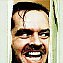First off, welcome to the forum. And second, man that's a tall order. Having no 3D experience you're in a world of trouble trying to draw a 4V geodesic dome.

I helped my son build a real geodesic dome out of paper last year for a school project. Here is a good link for those interested:

http://www.plunk.org/~grantham/public/geodesic_domes/geodesic_domes.html

I'm sure there are many ways of doing this. I bet one of the very talented people in this forum could even write a LISP program to create one on the fly. Heck a dome is just basic geometry. But to give step by step instructions and to someone with no 3D experience, that will take some doing.

If I have time I will try and work on something for you. But I will wager the other smarter folks in the forum will come up with a better solution. I saw a LISP program the other day which will plot all the points on a soccer ball, which is basically a geodesic sphere. If they can do that I'm sure someone could do it for a 4v dome.

Another quick google search and I found this:

Perhaps someone with more LISP knowledge than I could assist us here. This is a very old LISP routine which comes from 1988 apparently before ACAD brought in it's own GEO command. I have loaded this one but can't seem to run it with the command GEO nor ng3, etc... I am looking for another script if it's available.

My searches also turned up this one of our very own:

Edited by Bill Tillman

##### Share on other sites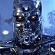You have an urgent need to both learn AutoCAD 3D and at the same time build this v4 geodesic dome? Would this happen to be a final project of some kind for school?

##### Share on other sitesI don't know if this OP gave up or what but I found a really cool site which has downloadable DXF files of different domes including a 4v model which he was looking for.

http://www.geo-dome.co.uk/article.asp?uname=3d_primatives

If you study one of these models, especially the 4v model you will find that building such a dome is not as easy as one would first think. And how one could reduce the process to a LISP program is daunting to think about. Still I hear it can be done. It's beyond my math skills for the moment.

Just in case this is a classroom assignment and one would certainly not want to turn in work which was merely copied from the net, here is a really cool tutorial on how to draft a dome, the hard way.

http://www.uni.edu/darrow/new/geodesic/drawings/draft.html

Laborious but still very educational and that's what it's all about.

Edited by Bill Tillman

##### Share on other sitesThx a liot for the help

##### Share on other sites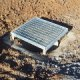Where would you typically use one of these domes? Are they simply an artistic roof structure in most instances?

##### Share on other sitesThe market is very limited. Domes have been around since antiquity and even the geometry to build them was discovered long ago. But I recall there was a boom for them in the 1970's when the Arab oil embargo happened but this was only short lived. I see a dome house every now and then and while they look unique I don't think there is enough asthetic appeal to them for the masses to latch on. And imagine a block where all the homes were domes, I think the neighborhood would be rather bland and have not much in flavor to be sellable.

Domes are used by the NOAA to cover their doppler radar stations, and of course you've got the big sports areneas which occassionaly place a dome in their design. They could be used for anything from greenhouses to just about anything but the market is not there and I think it has to do with just the basic egg shape that domes are. This is not a pleasing design to most people. Then you've got all your other considerations like windows, drywall...ever try to put a 4x8 sheet of plywood on a dome surface's interior. You end up cutting up a lot of waste and this is why builders hate them as well.

But a dome gives the largest area that can be covered with the least amount of material and they are very rigid. Still, if the public doesn't like the look, what have you got to sell?

##### Share on other sitesI realize that this is an old post, though I have come across a lisp for building a geodesic dome in ACAD. I had become rather interested in this topic and did a bit of researching. Reply to this post if you still want to know more. Regards!

##### Share on other sitesSure...I want to know more. Where is this lisp routine you speak of?

##### Share on other sitesIts a little rough but it works:

```
(defun negval (pnt / )
(mapcar '- pnt))

(defun vec->pnt (strt norm mag /)
(mapcar '+ strt (mapcar '(lambda (x) (* mag x))norm ))
)
(vec->pnt '(0 0 0) '(1 0 0) 1)

(defun normal (p /)
(mapcar '(lambda (i) (/ i (sqrt (apply '+ (mapcar '* p p))))) p))

(defun face (A B C n / ab ac bc)
(if (> n 0)
(progn
(setq AB (normal (mapcar '+ A B)))
(setq AC (normal (mapcar '+ A C)))
(setq BC (normal (mapcar '+ B C)))
(setq n (1- n))
(face A AB AC n)
(face B AB BC n)
(face C AC BC n)
(face AB AC BC n)
)
(progn
)
(vlax-3d-point (vec->pnt ctr (negval A) rad))
(vlax-3d-point (vec->pnt ctr (negval B) rad))
(vlax-3d-point (vec->pnt ctr (negval C) rad))
(vlax-3d-point (vec->pnt ctr (negval A) rad))
)
)
)
)

(defun c:geo8 ()
(if (> 0 (setq f (getint "\nEnter nth Order: ")))
(setq f (- f)))
(setq ctr (getpoint "\nSelect Center of Sphere: "))

(setq pntA '(0 0 1))
(setq pntB '(0 1 0))
(setq pntC '(1 0 0))
(face pntA pntB pntC f)
(command "array" (ssget "X" ) "" "p" ctr 4 "" "")
)
(defun c:geoico ()
(if (> 0 (setq f (getint "\nEnter nth Order: ")))
(setq f (- f)))
(setq ctr (getpoint "\nSelect Center of Sphere: "))

(setq x (sqrt (/ (+ 5.0 (sqrt 5.0)) 10.0))) ;these formulas calculate the
(setq y (sqrt (/ (- 3.0 (sqrt 5.0)) 10.0))) ;vertices of two icosahedral
(setq z (sqrt 0.2))                ;faces, the first having
(setq u (sqrt (/ (- 5.0 (sqrt 5.0)) 10.0))) ;vertices (0 0 1), (x y z),
(setq v (- (* x x)))               ;(u v z), and the second with
(setq p0 (list 0.0 0.0 1.0))       ;vertices (x y z), (u v z),
(setq p1 (list x y z))             ;(x -y -z).
(setq p2 (list u v z))
(setq p3 (list x (- y) (- z)))
(face p0 p1 p2 f)
(face p1 p2 p3 f)
(command "array" (ssget "X" ) "" "p" ctr 5 "" "")
)

```

This was created with help of a lisp that I found.

Each order increases the process time by a factor of four.

Order 0 = Basic Form

I haven't tried going higher than 5 on the Order.

Have fun!

##### Share on other sitesOk... So C:geoico isn't working quite right yet.... ((Hrm...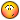) worked last night)

but C:geo8 is working correctly

ok all is working it was just a snaps issue

Edited by Hippe013
Snaps Issue

##### Share on other sitesThank you for sharing your lisp routine with us. I'm sure someone will find it handy. We have requests for help with geodesic domes a couple of times a year.

##### Share on other sitesThank you for sharing your lisp routine with us. I'm sure someone will find it handy. We have requests for help with geodesic domes a couple of times a year.

You're welcome! This was a really fun one to research! Helped me understand the whole recursive function thingy!

##### Share on other sitesYes, Hippe's code is good, but I had time to make some mods. to make the code even better (using of (ssget "_X") in DWG full with other entities is bad in my opinion)...

```(defun negval ( pnt )
(mapcar '- pnt)
)

(defun vec->pnt ( strt norm mag )
(mapcar '+ strt (mapcar '(lambda ( x ) (* mag x)) norm))
)

;;;(vec->pnt '(0 0 0) '(1 0 0) 1)

(defun normal ( p )
(mapcar '(lambda ( i ) (/ i (sqrt (apply '+ (mapcar '* p p))))) p)
)

(defun face ( A B C n / ab ac bc )
(if (> n 0)
(progn
(setq AB (normal (mapcar '+ A B)))
(setq AC (normal (mapcar '+ A C)))
(setq BC (normal (mapcar '+ B C)))
(setq n (1- n))
(face A AB AC n)
(face B AB BC n)
(face C AC BC n)
(face AB AC BC n)
)
(progn
)
(vlax-3d-point (vec->pnt ctr (negval A) rad))
(vlax-3d-point (vec->pnt ctr (negval B) rad))
(vlax-3d-point (vec->pnt ctr (negval C) rad))
(vlax-3d-point (vec->pnt ctr (negval A) rad))
)
)
)
)

(defun c:geo8 ( [b][color=red]/ *ms* f ctr rad pntA pntB pntC el ss[/color][/b] )
[b][color=red](setq *ms* (vla-get-block (vla-get-activelayout (vla-get-activedocument (vlax-get-acad-object)))))[/color][/b]
(if (> 0 (setq f (getint "\nEnter nth Order: ")))
(setq f (- f))
)
(setq ctr (getpoint "\nSelect Center of Sphere: "))
[b][color=red](initget 7)[/color][/b]
(setq pntA '(0 0 1))
(setq pntB '(0 1 0))
(setq pntC '(1 0 0))
[b][color=red]  (if (entlast)
(setq el (entlast))
)
(if el
(while (entnext el)
(setq el (entnext el))
)
)
[/color][/b]  (face pntA pntB pntC f)
[b][color=red]  (if (not el)
(progn
(setq el (entnext))
(while (setq el (entnext el))
)
)
(while (setq el (entnext el))
)
)
[/color][/b]  (command "_.ARRAY" [b][color=red]ss[/color][/b] "" "_P" [b][color=red]"_non"[/color][/b] ctr 4 "" "")
(princ)
)

(defun c:geoico ( [b][color=red]/ *ms* f ctr rad x y z u v p0 p1 p2 p3 el ss[/color][/b] )
[b][color=red](setq *ms* (vla-get-block (vla-get-activelayout (vla-get-activedocument (vlax-get-acad-object)))))[/color][/b]
(if (> 0 (setq f (getint "\nEnter nth Order: ")))
(setq f (- f))
)
(setq ctr (getpoint "\nSelect Center of Sphere: "))
[b][color=red](initget 7)[/color][/b]
(setq x (sqrt (/ (+ 5.0 (sqrt 5.0)) 10.0))) ;these formulas calculate the
(setq y (sqrt (/ (- 3.0 (sqrt 5.0)) 10.0))) ;vertices of two icosahedral
(setq z (sqrt 0.2))                ;faces, the first having
(setq u (sqrt (/ (- 5.0 (sqrt 5.0)) 10.0))) ;vertices (0 0 1), (x y z),
(setq v (- (* x x)))               ;(u v z), and the second with
(setq p0 (list 0.0 0.0 1.0))       ;vertices (x y z), (u v z),
(setq p1 (list x y z))             ;(x -y -z).
(setq p2 (list u v z))
(setq p3 (list x (- y) (- z)))
[b][color=red]  (if (entlast)
(setq el (entlast))
)
(if el
(while (entnext el)
(setq el (entnext el))
)
)
[/color][/b]  (face p0 p1 p2 f)
(face p1 p2 p3 f)
[b][color=red]  (if (not el)
(progn
(setq el (entnext))
(while (setq el (entnext el))
)
)
(while (setq el (entnext el))
)
)
[/color][/b]  (command "_.ARRAY" [b][color=red]ss[/color][/b] "" "_P" [b][color=red]"_non"[/color][/b] ctr 5 "" "")
(princ)
)
```

Regards, M.R.

##### Share on other sitesSince my sole purpose for testing was to check that Hippie013's lisp routine worked I started with a new drawing thus there were no other entities to be concerned about. None-the-less, thanks for the improvements. I'm sure someone, someday, will be thankful for the revised version.

##### Share on other sitesYes, Hippe's code is good, but I had time to make some mods. to make the code even better (using of (ssget "_X") in DWG full with other entities is bad in my opinion)...

Marko,

I appreciate the update to the code, and Yes, using (ssget "_X") was a poor choice. It is interesting to see my code from 5+ years ago and how different I would write the same code today. I would like to run tests with this code up against an updated version translated into vb.net and see if there is a significant performance change. Maybe if I find time I'll give the translation a go.

Till then, Cheers!

##### Share on other sitesHi Hippe, according to 3ds MAX software, it seems that there is also tetrahedral one, but it's ugly... Nevertheless I've coded according to your sub functions and for this one... Also I've rotated main points along X axis, so that now when geodesic sphere (tetrahedral) is created, you can just step in front view and slice it to hemisphere (dome)...

```(defun negval ( pnt )
(mapcar '- pnt)
)

(defun vec->pnt ( strt norm mag )
(mapcar '+ strt (mapcar '(lambda ( x ) (* mag x)) norm))
)

;;;(vec->pnt '(0 0 0) '(1 0 0) 1)

(defun normal ( p )
(mapcar '(lambda ( i ) (/ i (sqrt (apply '+ (mapcar '* p p))))) p)
)

(defun face ( A B C n / ab ac bc )
(if (> n 0)
(progn
(setq AB (normal (mapcar '+ A B)))
(setq AC (normal (mapcar '+ A C)))
(setq BC (normal (mapcar '+ B C)))
(setq n (1- n))
(face A AB AC n)
(face B AB BC n)
(face C AC BC n)
(face AB AC BC n)
)
(progn
)
(vlax-3d-point (vec->pnt ctr (negval A) rad))
(vlax-3d-point (vec->pnt ctr (negval B) rad))
(vlax-3d-point (vec->pnt ctr (negval C) rad))
(vlax-3d-point (vec->pnt ctr (negval A) rad))
)
)
)
)

(defun face-nomirror ( A B C n / ab ac bc )
(if (> n 0)
(progn
(setq AB (normal (mapcar '+ A B)))
(setq AC (normal (mapcar '+ A C)))
(setq BC (normal (mapcar '+ B C)))
(setq n (1- n))
(face-nomirror A AB AC n)
(face-nomirror B AB BC n)
(face-nomirror C AC BC n)
(face-nomirror AB AC BC n)
)
(progn
)
)
)
)

(defun c:geo4-tetra ( / *ms* f ctr rad p0 p1 p2 p3 )
(setq *ms* (vla-get-block (vla-get-activelayout (vla-get-activedocument (vlax-get-acad-object)))))
(if (> 0 (setq f (getint "\nEnter nth Order: ")))
(setq f (- f))
)
(setq ctr (getpoint "\nSelect Center of Sphere: "))
(initget 7)
;|
(setq p0 (normal (list 0.0 0.0 (/ (sqrt 3.0) 2.0))))
(setq p1 (normal (list 0.0 (/ (sqrt 2.0) (sqrt 3.0)) (/ (sqrt 3.0) (- 6.0)))))
(setq p2 (normal (list (/ (sqrt 2.0) (- 2.0)) (/ (- 1.0) (sqrt 6.0)) (/ (sqrt 3.0) (- 6.0)))))
(setq p3 (normal (list (/ (sqrt 2.0) 2.0) (/ (- 1.0) (sqrt 6.0)) (/ (sqrt 3.0) (- 6.0)))))
|;
(setq p0 (trans (polar '(0.0 0.0 0.0) (+ (* 0.5 pi) (atan (/ (sqrt 6.0) 3.0) (/ (sqrt 3.0) 3.0))) 1.0) '(1.0 0.0 0.0) 0))
(setq p1 (trans (polar '(0.0 0.0 0.0) (+ (- (atan (/ (sqrt 3.0) 6.0) (/ (sqrt 2.0) (sqrt 3.0)))) (atan (/ (sqrt 6.0) 3.0) (/ (sqrt 3.0) 3.0))) 1.0) '(1.0 0.0 0.0) 0))
(setq p2 (trans (polar (list 0.0 0.0 (/ (sqrt 2.0) (- 2.0))) (+ (+ pi (atan (/ (sqrt 3.0) 6.0) (/ 1.0 (sqrt 6.0)))) (atan (/ (sqrt 6.0) 3.0) (/ (sqrt 3.0) 3.0))) (/ (sqrt 3.0) 3.0)) '(1.0 0.0 0.0) 0))
(setq p3 (trans (polar (list 0.0 0.0 (/ (sqrt 2.0) 2.0)) (+ (+ pi (atan (/ (sqrt 3.0) 6.0) (/ 1.0 (sqrt 6.0)))) (atan (/ (sqrt 6.0) 3.0) (/ (sqrt 3.0) 3.0))) (/ (sqrt 3.0) 3.0)) '(1.0 0.0 0.0) 0))
(face-nomirror p0 p1 p2 f)
(face-nomirror p1 p2 p3 f)
(face-nomirror p2 p3 p0 f)
(face-nomirror p0 p1 p3 f)
(princ)
)

(defun c:geo8-octa ( / *ms* f ctr rad pntA pntB pntC el ss )
(setq *ms* (vla-get-block (vla-get-activelayout (vla-get-activedocument (vlax-get-acad-object)))))
(if (> 0 (setq f (getint "\nEnter nth Order: ")))
(setq f (- f))
)
(setq ctr (getpoint "\nSelect Center of Sphere: "))
(initget 7)
(setq pntA '(0 0 1))
(setq pntB '(0 1 0))
(setq pntC '(1 0 0))
(if (entlast)
(setq el (entlast))
)
(if el
(while (entnext el)
(setq el (entnext el))
)
)
(face pntA pntB pntC f)
(if (not el)
(progn
(setq el (entnext))
(while (setq el (entnext el))
)
)
(while (setq el (entnext el))
)
)
(command "_.ARRAY" ss "" "_P" "_non" ctr 4 "" "")
(princ)
)

(defun c:geo20-icosa ( / *ms* f ctr rad x y z u v p0 p1 p2 p3 el ss )
(setq *ms* (vla-get-block (vla-get-activelayout (vla-get-activedocument (vlax-get-acad-object)))))
(if (> 0 (setq f (getint "\nEnter nth Order: ")))
(setq f (- f))
)
(setq ctr (getpoint "\nSelect Center of Sphere: "))
(initget 7)
(setq x (sqrt (/ (+ 5.0 (sqrt 5.0)) 10.0)))
(setq y (sqrt (/ (- 3.0 (sqrt 5.0)) 10.0)))
(setq z (sqrt 0.2))
(setq u (sqrt (/ (- 5.0 (sqrt 5.0)) 10.0)))
(setq v (- (* x x)))
(setq p0 (list 0.0 0.0 1.0))
(setq p1 (list x y z))
(setq p2 (list u v z))
(setq p3 (list x (- y) (- z)))
(if (entlast)
(setq el (entlast))
)
(if el
(while (entnext el)
(setq el (entnext el))
)
)
(face p0 p1 p2 f)
(face p1 p2 p3 f)
(if (not el)
(progn
(setq el (entnext))
(while (setq el (entnext el))
)
)
(while (setq el (entnext el))
)
)
(command "_.ARRAY" ss "" "_P" "_non" ctr 5 "" "")
(princ)
)
```

Here is complete code, but I've also noticed that when tetrahedral geodesic sphere is created, some faces are inside it - those just around main vertices of tetrahedron... [EDIT : I think I've corrected this issue - sub function wasn't recursive to itself but it called (face) instead (face-nomirror)]

Regards, and I hope that my intervention is also useful...

M.R.

Edited by marko_ribar
code corrected...

##### Share on other sitesI want to try this GEO routine, but I doesn't seem te be working... I hope someone can help me?

When I load the lisp and run either geo8 or geoici, I get a 'too few arguments' error. (running autocad 2017)

I am not very famillia﻿r with lisp's, so perhaps I am forgetting something?

Lots of thanks from the Netherlands,

##### Share on other sites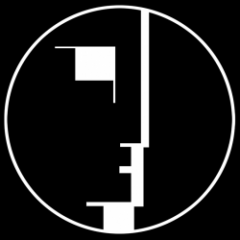10 hours ago, artyvisual said:

I want to try this GEO routine, but I doesn't seem te be working... I hope someone can help me?

Which routine are you using? Try the one that Hippe013 posted. It works for me in 2018 with no errors.

##### Share on other sites...Yes, thank you. I tried the latest version of Marko, and I got the error, but the one Hippe poste is indeed working. Thanks

## Join the conversation

You can post now and register later. If you have an account, sign in now to post with your account.
Note: Your post will require moderator approval before it will be visible.×   Pasted as rich text.   Paste as plain text instead

Only 75 emoji are allowed.